Next Article in Journal
Denitrification Control in a Recirculating Aquaculture System—A Simulation Study
Next Article in Special Issue
Effect of Rotor Spacing and Duct Diffusion Angle on the Aerodynamic Performances of a Counter-Rotating Ducted Fan in Hover Mode
Article

# Thermal Performance of T-shaped Obstacles in a Solar Air Heater

1
Department of Mechanical Engineering, Graduate School, Inha University, Incheon 22212, Korea
2
Department of Mechanical Engineering, Inha University, Incheon 22212, Korea
*
Author to whom correspondence should be addressed.
Processes 2020, 8(10), 1305; https://doi.org/10.3390/pr8101305
Received: 18 September 2020 / Revised: 7 October 2020 / Accepted: 13 October 2020 / Published: 17 October 2020

## Abstract

This paper proposes T-shaped ribs as obstacles attached to the heat absorber plate in a rectangular solar air heater to promote heat transfer. The thermal and aerodynamic performance of the solar heater was numerically evaluated using three-dimensional Reynolds-averaged Navier–Stokes equations with the shear stress transport turbulence model. A parameter study was performed using the ratios of rib height to channel height, rib width to channel width, and rib width to rib height. The area-averaged Nusselt number and friction factor were selected as the performance parameters of the solar air heater to evaluate the heat transfer and friction loss, respectively. In addition, the performance factor was defined as the ratio of the area-averaged Nusselt number to the friction factor. The maximum area-averaged Nusselt number was found at h/e = 0.83 for a fixed rib area. Compared with triangular ribs, the T-shaped ribs showed up to a 65 % higher area-averaged Nusselt number and up to a 49.7% higher performance factor.

## 1. Introduction

Recently, as the problem of environmental pollution due to the use of fossil fuels has emerged, interest in solar heat systems as a renewable energy source is increasing. A solar air heater (SAH) is a device that heats air flowing over an absorber plate by using solar energy. It is used for space heating, drying of agricultural products, and dehydration of industrial products .
Compared to liquid solar heaters, SAHs have a disadvantage of low heat transfer rate, because the density of air is lower than that of a liquid. The heat transfer performance of an SAH is determined by various factors such as the velocity of the flow, the length and depth of the heater, and the shape of the heat absorber plate. The ratio between the area of the actual heat absorber and the absorber area normal to the solar radiation, which is called the absorber shape factor, is an important parameter in the design of SAHs.
Obstacles attached to the heat absorber plate are generally known to increase the heat transfer by enlarging the heat transfer area and enhancing turbulence intensity. However, as the area of the obstacles attached to the heat absorber plate increases, the pressure drop through the SAH also increases. Therefore, many studies have been conducted on the shape of the obstacles to enhance the overall heat transfer performance by increasing the heat transfer while minimizing the pressure drop [2,3,4,5,6,7,8,9,10,11,12,13,14].
Kabeel and Mecarik  studied the effect of the shape of the heat absorber plate on the performance of an SAH. It was confirmed that the heat transfer performance of the SAH with triangular obstacles was higher than that with longitudinal pins. Moummi et al.  performed an energy analysis of an SAH with rectangular plate fins. The results for the heat transfer coefficient were compared with the results obtained for the SAH without obstacles. The collector efficiency factor of the SAH with plate pins increased by 30% compared to the case without obstacles. Essen  performed energy and exergy analysis through experiments on obstacles of various shapes attached to an SAH. He concluded that the heat and exergy efficiencies of the SAH with obstacles increased as compared to the SAH without obstacles and the collector efficiency factor depended on the shape, area, orientation, and arrangements of the obstacles. Saini and Saini  conducted an experimental study on the effect of the rib shape on the heat transfer performance of an SAH with arc-shaped ribs. They developed correlations for the Nusselt number and friction factor in terms of Reynolds number (Re), relative roughness height, and arc angle of ribs.
Ozgen et al.  conducted an efficiency evaluation of an SAH with the flows flowing over the upper and lower surfaces of a heat absorber plate where aluminum cans were attached. Depaiwa et al.  experimentally studied the forced convective heat transfer and friction loss for the turbulent flow in an SAH with rectangular winglet vortex generators. They tested an SAH with 20 winglet vortex generators at Reynolds numbers ranging from 5000 to 23,000. The heat transfer rates in this SAH were 174% to 182% higher than that of the SAH with a smooth absorber plate. Bekele et al.  performed an experimental and numerical analysis on the effects of the height and longitudinal pitch of triangular obstacles on the heat transfer in an SAH. Through the analysis of the internal flow of the SAH, it was reported that recirculating flows occurring around the triangular obstacles effectively increased the turbulence intensity, thereby increasing the heat transfer rate by 3.6 times compared to the SAH without obstacles. Yadav et al.  conducted an experimental study on the heat transfer performance and friction factor of the turbulent flow over a heat absorber plate with circular protrusions arranged in angular arc. The Nusselt number and friction factor were found to be 2.89 times and 2.93 times higher than those of the unobstructed SAH, respectively.
Kulkarni and Kim  performed a numerical analysis to find the optimal shape of obstacles attached to an SAH. Numerical analysis was performed for four different shapes and three different arrangements of the obstacles. The Nusselt number and friction coefficient were greatly influenced by the shape and arrangement of the obstacles, and pentagonal obstacles showed the highest performance factor among the tested shapes. Alam and Kim  performed a numerical analysis to predict the heat transfer performance of an SAH with conical protrusion ribs. The maximum thermal efficiency of the collector was reported to be 69.8%. They developed correlations for the Nusselt number and friction factor in terms of Reynolds number, relative roughness height, and relative rib pitch. Compared with the results of numerical analysis, the correlations for the Nusselt numbers and friction factor showed average absolute standard deviations of 2.78% and 5.25%, respectively. In addition, studies on SAHs with various types of obstacles such as V-shaped ribs, diamond-shaped ribs, and arc-shaped ribs have been also conducted to date [12,13,14].
In this study, T-shaped ribs are newly proposed to further improve the performance of an SAH by considering both the heat transfer and pressure drop. The heat transfer and pressure drop characteristics of the SAH with ribs were analyzed using three-dimensional Reynolds-averaged Navier–Stokes (RANS) equations. A parametric study was conducted to confirm the effects of geometric parameters of the T-shaped ribs on the heat transfer and pressure drop in the SAH. The performance of the SAH with the proposed ribs was compared to SAHs with previously developed obstacles.

## 2. Solar Air Heater Model

Figure 1 shows the computational domain of the SAH and geometric parameters of the T-shaped rib. Only a half of the entire SAH domain is included in the computational domain using symmetric conditions. The computational domain consists of three sections; inlet section (800 mm × 150 mm × 50 mm (L1 × W/2 × H)), test section (1200 mm × 150 mm × 50 mm (L2 × W/2 × H)), and outlet section (500 mm × 150 mm × 50 mm (L3 × W/2 × H)). This computational domain for evaluating the performance of the SAH was set according to the guidelines of the ASHRAE standard 93–97 , and the lengths of the inlet and outlet sections of the domain are 5(WH)0.5 and 2.5(WH)0.5, respectively.
The upper wall (i.e., heat absorber plate) of the test section absorbs solar energy, and air is heated as it flows from the inlet to the outlet of the channel. The obstacles, T-shaped ribs, are attached to the heat absorber plate. Thirteen rows of ribs are arranged in a zigzag, as shown in Figure 1a. The thickness of the rib is 0.5 mm, and t is 8 mm. Each row contains one or one and a half ribs in the computational domain. The first row of ribs is located 71.75 mm downstream of the inlet of the test section.

## 3. Numerical Methods

In this study, ANSYS-CFX 15.0 , a commercial code which employs an unstructured grid, was used to analyze the flow and heat transfer using RANS equations. The shear stress transport (SST)  model was used for the analysis of turbulence. The SST model was designed to take advantage of the k-ε and k-ω models by combining these two models using a weighting function so that the k-ω model is applied near the wall and the k-ε model is applied in the other area. The SST model is known to be effective in predicting the flow recirculation due to separation.
Air at 25 °C was used as the working fluid, and velocity and static pressure conditions were assigned to the inlet and outlet boundaries, respectively. A constant heat flux condition of 815 W/m2 was applied to the surface of the heat absorber plate, and the other solid surfaces were assumed to be adiabatic. The symmetric conditions were used at the symmetric plane of the computational domain, and a no-slip condition was used at the wall boundaries. An unstructured tetrahedral grid system was constructed in most of the computational domain, but prism meshes were placed near the wall to resolve the laminar sublayer. In order to use low-Re modeling for near-wall turbulence, the y+ values of the first grid points from the wall were kept at less than 1.0.
To find the grid dependency of the numerical solution, a test was performed according to the procedure proposed by Roache  and Celik and Karatekin . This test analyzes the grid convergence index (GCI), which represents numerical uncertainty through the estimation of discretization error based on Richardson extrapolation. Table 1 shows the results of the GCI analysis where the grid refinement factor (r) was set to 1.3 for three different grid systems (N1, N2, and N3). This test was performed on the SAH with the reference ribs described in Table 2. As the number of grid nodes increases, the Nusselt number tends to converge gradually. When N2 is used, the extrapolated relative error ($e e x t 21$) is 0.0015% and the relative error is 0.0019%, which confirms a relatively small numerical uncertainty. Therefore, based on this result, the optimum grid system is selected as N2, which is shown in Figure 2.
In order to determine the convergence of the numerical solution, the root mean square residual was reduced to 10−6 or less, and about 1200 iterations were performed. The computational time required for a single analysis was about 14 h when a personal computer with an Intel Core i7 3.41 GHz CPU was used for the computations.

## 4. Geometric and Performance Parameters

In order to examine the effects of the rib shape shown in Figure 1 on the heat transfer performance and pressure drop, three dimensionless parameters were selected; the ratios of the rib height to the channel height (h/H), the rib width to the channel width (e/W), and the rib height to the rib width (h/e). The ranges and reference values of these parameters are shown in Table 2.
Three performance parameters are defined to evaluate the heat transfer and pressure drop in the SAH. The performance parameter related to the heat transfer is defined as follows:
$F N u = N u o N u s$
Nuo is the area-averaged Nusselt number on the heat transfer surface.
$N u o = q o D h k a ( T p − T s )$
where Tp is the average temperature at the surface of the heat absorber plate including the obstacles, Ts is the bulk temperature of the working fluid, ka is the thermal conductivity of the fluid, qo is the heat flux at the heat absorber plate, Dh is the hydraulic diameter of the flow channel, and Nus is the Nusselt number for a fully developed flow in a smooth channel without obstacles, which is calculated from the following Dittus–Boelter equation.
$N u s = 0.024 R e 0.8 P r 0.4$
where Reynolds number, Re, is defined using a hydraulic diameter, and Pr indicates the Prandtl number.
The definition of the performance parameter related to the pressure drop is as follows:
$F f = ( f o f s ) 1 / 3$
Here, fo is the friction factor in the flow channel with obstacles.
$f o = 2 ( Δ P ) D h 4 ρ L U 2$
where ∆p is the pressure drop in the test section, ρ is the density of the working fluid, U is the average velocity of the flow, L is the length of the flow channel (test section), and fs is the friction factor for the fully developed flow in the smooth channel, which is calculated from the following modified Blasius equation.
$f s = 0.085 R e − 0.25$
The performance factor, PF , for evaluating the performance of an SAH considering both the heat transfer performance and pressure drop at the same time, is defined as follows:

## 5. Results and Discussion

To prove the validity of the numerical analysis, the numerical results for the Nusselt number, Nuo, and friction factor, fo, are compared with corresponding empirical formulas for different Reynolds numbers in the flow channel without obstacles, as shown in Figure 3. The numerical results show good overall agreement with the empirical formulas. As Reynolds number increases, the relative error tends to decrease in the case of Nuo. Compared with the experimental data, the average relative error of the computed Nusselt numbers within the tested Reynolds number range is less than 3.29%, and it is less than 2.71% for the friction factor.
In addition, the validation test was also performed for the case with obstacles. The numerical results are compared with the experimental data obtained by Bekele et al.  for the SAH with triangular obstacles under the same boundary conditions, as shown in Figure 4. The numerical results agree well qualitatively with the experimental data. In the results for the Nusselt number, the computational and empirical slopes according to the Reynolds number are exactly the same but, quantitatively, they show a small difference unlike the case without obstacles. The average relative error for the Nusselt number compared to the experimental data within the tested Reynolds number range is less than 4.43%, and that for the friction factor is less than 1.94%, which indicates that the accuracy of the numerical analysis results is acceptable for further calculations.
The three dimensionless geometric parameters of the SAH with proposed T-shaped ribs presented in Table 2 were used to find the effects of the rib shape on the performance parameters, i.e., FNu, Ff, and PF. The parametric study was performed at a Reynolds number of 22,600. In the case of two variables, h/H and e/W, the reference value in Table 2 was applied to the parameter that was not changed, and the area of the rib was changed accordingly during the parametric study. However, in the case of h/e, the test was conducted with the rib area fixed at 375 mm2. Therefore, in this case, both the absolute values of h and e changed to keep the area constant according to the change of h/e. In all cases, the size of t was kept constant.
The results of the parametric study for the ratio of the rib height to the channel height (h/H) are shown in Figure 5, Figure 6, Figure 7, Figure 8 and Figure 9. Figure 5 and Figure 6 show the changes in the performance parameters, FNu and Ff, defined by Equations (1) and (2), respectively, in a range of 0.3 ≤ h/H ≤ 0.7. The computational values are presented at five points indicated by circular symbols, and the line is a curve fit of the values at these points. It can be seen that FNu has the maximum value between h/H = 0.5 and 0.6 and it gradually decreases after that (Figure 5). On the other hand, Ff continues to increase as h/H increases, as shown in Figure 6. Therefore, as the rib height increases with the fixed rib width, there exists the maximum value in the heat transfer rate, but the pressure drop increases continuously. Similar heat transfer performances are found at h/H = 0.5 and 0.6 (Figure 5), but the pressure drop is lower at the lower rib height (h/H = 0.5), as shown in Figure 6. Therefore, in the PF distribution shown in Figure 7, the maximum performance factor appears at h/H = 0.5.
Figure 8 shows the streamlines between the 5th and 7th ribs (indicated by a dark region in Figure 1b) at the symmetric plane (i.e., x-y plane at z = 0) for different h/H. Due to the geometric shape of the rib, two recirculation regions are formed in the direction of the rib height. As h/H increases, the size of the recirculation region near the upper wall increases. Additionally, as h/H increases from 0.3 to 0.5, the reattachment distance rapidly decreases. This rapid reduction in the reattachment distance in this h/H range greatly enhances the heat transfer, as shown in Figure 5, due to early re-development of the thermal boundary layer. In Figure 9, the local Nusselt number distributions on the heat transfer surface for h/H = 0.3 and 0.5 are different. In the case of h/H = 0.5, the Nusselt number level appears to be higher around and downstream of each rib compared to the case of h/H = 0.3.
Figure 10, Figure 11, Figure 12, Figure 13 and Figure 14 show the effects of the ratio of the rib width to the channel width (e/W) on the performance of the SAH. Figure 10 and Figure 11 show the variations of the two performance parameters, FNu and Ff, respectively, with e/W, in a range of 0.067 ≤ e/W ≤ 0.133. As e/W increases, both FNu and Ff increase almost linearly. This is presumed to be a phenomenon that occurs because both the turbulence intensity and pressure loss increase due to the increase in the area of the obstacles as e increases while the height h is kept constant. In the PF distribution shown in Figure 12, the maximum value is found around e/W = 0.08, but the overall variation with e/W is very small compared to that in Figure 7 for h/H.
Figure 13 shows the streamlines between the 5th and 7th ribs at the symmetric plane for different h/H. It can be seen that as e/W increases, the recirculation region located near the top of the rib increases. Since the reattachment distance does not change with e/W, the heat transfer enhancement according to the change in e/W does not seem to be related to the reattachment distance. Figure 14 shows the distributions of the Nusselt number on the heat transfer surface between the 5th and 7th ribs. As e/W increases, the increased width of the rib increases the width of the high Nusselt number area in the lateral direction, thereby increasing the overall Nusselt number.
The variation of FNu with the ratio of the rib height to the rib width (h/e) keeping the rib area constant is shown in Figure 15. At h/e = 0.83, the heat transfer is maximized. This variation of FNu is similar to the variation with h/H shown in Figure 5. However, unlike Figure 5, the variation curve of FNu shown in Figure 15 is more symmetrical as the area of the rib is kept constant in this case. The variation of Ff with h/e shown in Figure 16 is very small compared to the variations with h/H and e/W shown in Figure 6 and Figure 11, respectively. In the cases with h/H and e/W, the area of the rib changes, but in this case with h/e, the area of the rib remains constant. Accordingly, it can be seen that the pressure drop is greatly influenced by the area of the rib rather than the aspect ratio of the rib.
Since the change in the pressure drop appears small over the entire range of h/e, the variation of the performance factor PF with h/e, shown in Figure 17, shows a qualitatively similar variation to that of FNu (Figure 15). The maximum value of PF also appears at h/e = 0.83.
Figure 18 shows the Nusselt number distributions on the heat transfer surface between the 5th and 7th rib rows. At h/e = 0.83, where the highest heat transfer occurs, the area of the high Nusselt number region is the largest. This indicates that the heat transfer enhancement due to the production of turbulent kinetic energy becomes most effective when h and e have similar sizes while keeping the rib area constant.
To demonstrate the superiority of the heat transfer performance of the SAH with T-shaped ribs proposed in this study, the performance parameters are compared with the experimental data for the SAH with triangular ribs measured by Bekele et al. , as shown in Figure 19 and Figure 20. The comparison was performed for the same cross-sectional area of the ribs and the same spacing between the ribs. The T-shaped rib used for the comparison is the reference rib presented in Table 2. Figure 19 shows the variations of the Nusselt number (Nuo) and friction factor (fo) with Reynolds number. In the case of the friction factor, the T-shaped ribs show a slightly larger value than that with the triangular ribs in the entire Reynolds number range. However, the Nusselt number shows a large uniform improvement at all Reynolds numbers. Compared to the triangular ribs, the T-shaped ribs show Nusselt numbers that are 65% higher at Re = 4510 and 21.5% higher at Re = 22,600. On the other hand, a 35% higher pressure drop occurs at Re = 4510 and a 31% higher pressure drop occurs at Re = 22,600 than for the triangular ribs. Figure 20 shows the comparison of the performance factor PF between the two different ribs. The PF of the T-shaped ribs is 49.7% higher at Re = 4510, and 11% higher at Re = 22,600 than for the triangular ribs.
Table 3 shows a comparison of PF ranges among various obstacle shapes developed to date. These obstacles were tested by previous experimental or numerical studies using the parameters of longitudinal pitch (Pl/e), relative obstacle height (e/H), relative roughness height (e/D), relative roughness pitch (P/e), relative longitudinal pitch between the rows of winglets (P/H), and number of waves on the delta winglet (ϕ). The T-shaped ribs proposed in this study cause two recirculation regions formed in the height direction, as shown in Figure 8 and Figure 13, due to their unique geometric shape. These multiple recirculation zones are expected to promote the production of turbulent kinetic energy and thus further enhance the turbulent heat transfer compared to the other obstacles in Table 3.

## 6. Conclusions

In this study, T-shaped ribs with a staggered arrangement are proposed to enhance the heat transfer in an SAH, and the effects of the geometric parameters of the ribs on the thermal and aerodynamic performance of the SAH were analyzed using RANS equations. The numerical results for the heat transfer rate and friction factor were validated by comparing with experimental data for the smooth duct and the SAH with triangular obstacles. In the case of the SAH with the obstacles, the average relative error of the average Nusselt number between the numerical and experimental results was less than 4.43% within the tested Reynolds number range, and that of the friction factor was less than 1.94%. In a parametric study using the ratios of the rib height to the channel height (h/H) and the rib width to the channel width (e/W), the performance factor (PF) was far more sensitive to h/H than to e/W, and the maximum PF was found at h/H = 0.5 for a fixed e/W. When h/e was varied by keeping the rib area constant, the variation of the friction factor with h/e was very small due to the fixed rib area, and thus the variation of the average Nusselt number was qualitatively similar to that of PF and their maximum values were commonly found at h/e = 0.83. The proposed T-shaped ribs showed superior performance to several other heat-transfer enhancement obstacles developed to date. For example, when compared with the triangular ribs, the T-shaped ribs showed a 49.7% higher PF at a Reynolds number of 4510, and an 11% higher PF at a Reynolds number of 22,600. To generalize the present results for staggered T-shaped obstacles, further studies are required for the SAH using different arrangements of T-shaped obstacles.

## Author Contributions

S.-Y.A. presented the main idea of the T-shaped ribs; S.-Y.A. and K.-Y.K. contributed to the overall composition and writing of the manuscript; S.-Y.A. performed numerical analysis and analyzed the data; K.-Y.K. revised and finalized the manuscript. All authors have read and agreed to the published version of the manuscript.

## Funding

This work was supported by a National Research Foundation of Korea (NRF) grant funded by the Korean government (MSIT) (No. 2019R1A2C1007657).

## Conflicts of Interest

The authors declare there is no conflict of interest.

## References

1. Varun Saini, R.P.; Singal, S.K. A review on roughness geometry used in solar air heaters. Sol. Energy 2007, 81, 1340–1350. [Google Scholar] [CrossRef]
2. Kabeel, A.E.; Mecarik, K.K. Shape optimization for absorber plates of solar air collector. Renew. Energy 1998, 13, 121–131. [Google Scholar] [CrossRef]
3. Moummi, N.; Ali, S.Y.; Moummi, A.; Desmons, J.Y. Energy analysis of a solar air collector with rows of fins. Renew. Energy 2004, 29, 2053–2064. [Google Scholar] [CrossRef]
4. Esen, H. Experimental energy and exergy analysis of a double-flow solar air heater having different obstacles on absorber plates. Build. Environ. 2008, 43, 1046–1054. [Google Scholar] [CrossRef]
5. Saini, S.K.; Saini, R.P. Development of correlations for Nusselt number and friction factor for solar air heater with roughened duct having arc-shaped wire as artificial roughness. Sol. Energy 2008, 82, 1118–1130. [Google Scholar] [CrossRef]
6. Ozgen, F.; Esen, M.; Esen, H. Experimental investigation of thermal performance of a double-flow solar air heater having aluminium cans. Renew. Energy 2009, 34, 2391–2398. [Google Scholar] [CrossRef]
7. Depaiwa, N.; Chompookham, T.; Promvonge, P. Thermal enhancement in a solar air heater channel using rectangular winglet vortex generators. In Proceedings of the PEA-AIT International Conference on Energy and Sustainable Development: Issues and Strategies, Chiang Mai, Thailand, 2–4 June 2010; pp. 1–5. [Google Scholar]
8. Bekele, A.; Mishra, M.; Dutta, S. Heat transfer augmentation in solar air heater using delta shaped obstacle mounted on the absorber plate. Int. J. Sustain. Energy 2013, 32, 53–69. [Google Scholar] [CrossRef]
9. Yadav, S.; Kaushal, M.; Varun, S. Nusselt number and friction factor correlations for solar air heater duct having protrusions as roughness elements on absorber plate. Exp. Thermal Fluid Sci. 2013, 44, 34–41. [Google Scholar] [CrossRef]
10. Kulkarni, K.; Kim, K.Y. Comparative study of solar air heater performance with various shapes and configurations of obstacles. Heat Mass Transf. 2016, 52, 2795–2811. [Google Scholar] [CrossRef]
11. Alam, T.; Kim, M.H. Heat transfer enhancement in solar air heater duct with conical protrusion roughness ribs. Appl. Therm. Eng. 2017, 126, 458–469. [Google Scholar] [CrossRef]
12. Kumar, A.; Saini, R.P.; Saini, J.S. Development of correlations for Nusselt number and friction factor for solar air heater with roughened duct having multi v-shaped with gap rib as artificial roughness. Renew. Energy 2013, 58, 151–163. [Google Scholar] [CrossRef]
13. Pawar, S.S.; Hindolia, D.A.; Bhagoria, J.L. Experimental study of Nusselt number and Friction factor in solar air heater duct with diamond shaped rib roughness on absorber plate. Am. J. Eng. Res. 2013, 2, 60–68. [Google Scholar]
14. Singh, A.P.; Varun, S. Heat transfer and friction factor correlation for multiple arc shape roughness elements on the absorber plate used in solar air heaters. Exp. Therm. Fluid. Sci. 2014, 54, 117–126. [Google Scholar] [CrossRef]
15. ASHRAE. Method of Testing to Determine the Thermal Performance of Solar Collectors; Standard 93–97; Refrigeration and Air Conditioning Engineering: New York, NY, USA, 1997. [Google Scholar]
16. ANSYS. ANSYS CFX-Solver Theory Guide-Release 15.0.; ANSYS, Inc.: Canonsburg, Pennsylvania, 2013. [Google Scholar]
17. Bardina, J.E.; Huang, P.; Coakley, T. Turbulence Modeling Validation. AIAA J. Spacecr. Rocket. 1997, 40, 313–325. [Google Scholar]
18. Roache, P.J. Verification of Codes and Calculations. AIAA J. 1998, 36, 696–702. [Google Scholar] [CrossRef]
19. Celik, I.; Karatekin, O. Numerical Experiments on Application of Richardson Extrapolation with Nonuniform Grids. ASME J. Fluids 1997, 119, 584–590. [Google Scholar] [CrossRef]
20. Webb, R.I.; Eckert, E.R.G. Application of rough surface to heat exchanger design. Int. J. Heat. Mass Transf. 1972, 15, 1647–1658. [Google Scholar] [CrossRef]
21. Yadav, A.S.; Bhagoria, J.L. A CFD based thermo-hydraulic performance analysis of an artificially roughened solar air heater having equilateral triangle sectioned rib roughness on the absorber plate. Int. J. Heat Mass Transf. 2014, 70, 1016–1039. [Google Scholar] [CrossRef]
22. Saini, N.S.; Deo, S.; Chander, J.S. Performance analysis of solar air heater duct roughened with multigap V-down ribs combined with staggered ribs. Renew. Energy 2016, 91, 484–500. [Google Scholar]
23. Chamoli, V.B.; Gawande, A.S.; Dhoble, D.B.; Zodpe, S. Experimental and CFD investigation of convection heat transfer in solar air heater with reverse L-shaped ribs. Sol. Energy 2016, 131, 275–295. [Google Scholar]
24. Chamoli, J.S.; Sawhney, R.; Maithani, S. Experimental investigation of heat transfer and friction factor characteristics of solar air heater using wavy delta winglets. Appl. Therm. Eng. 2017, 117, 740–751. [Google Scholar]
Figure 1. Computational domain of the solar air heater with T-shaped ribs. (a) Computational domain, (b) Test section.
Figure 1. Computational domain of the solar air heater with T-shaped ribs. (a) Computational domain, (b) Test section.Figure 2. Example of grid system.
Figure 2. Example of grid system.Figure 3. Validation of numerical results for smooth duct.
Figure 3. Validation of numerical results for smooth duct.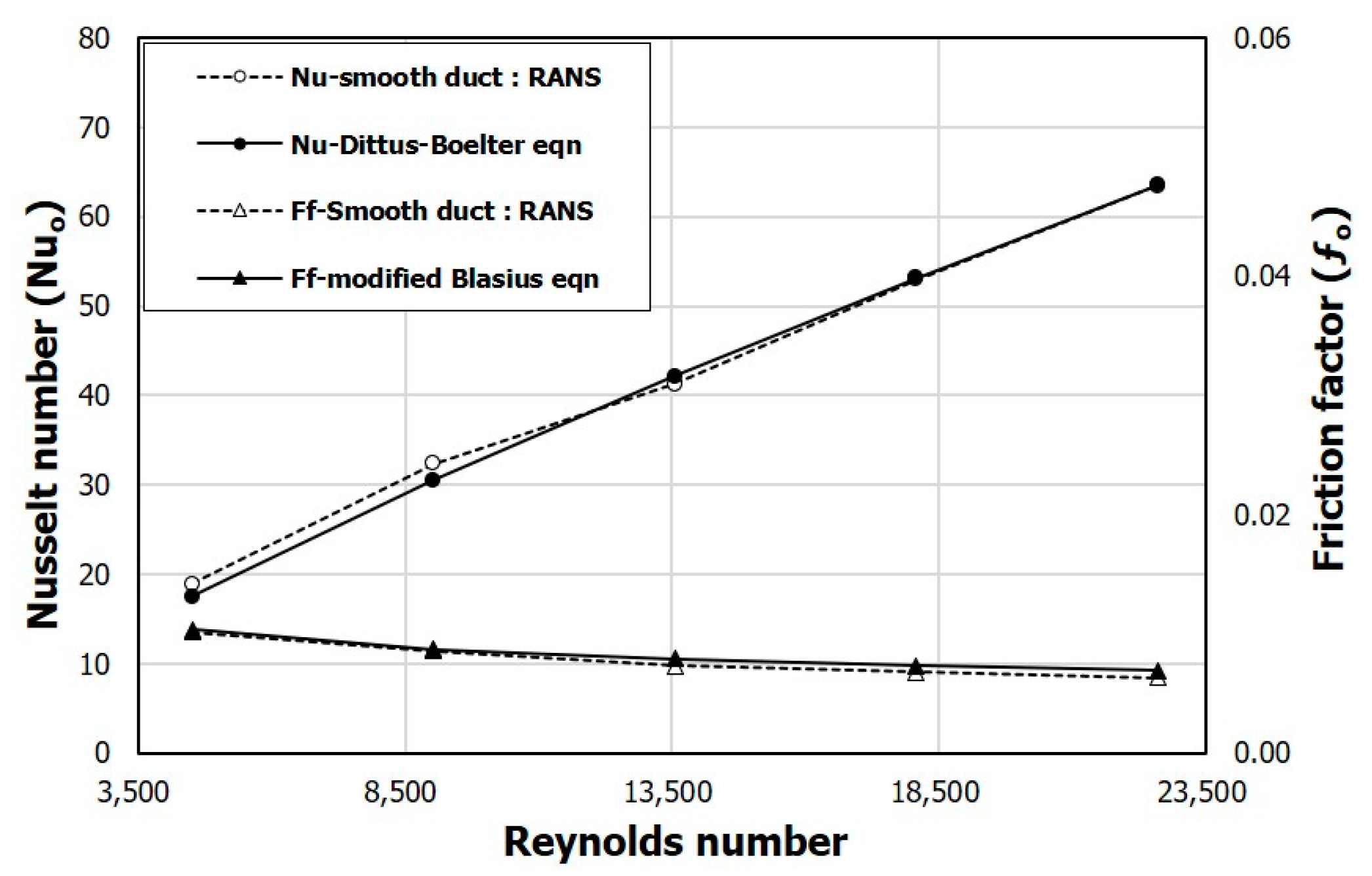Figure 4. Validation of numerical results for a solar air heater (SAH) with triangular ribs using experimental data of Bekele et al. .
Figure 4. Validation of numerical results for a solar air heater (SAH) with triangular ribs using experimental data of Bekele et al. .Figure 5. Variation of the performance parameter related to heat transfer (FNu) with the ratio of the rib height to the channel height (h/H) (the ratio of the rib width to the channel width (e/W) = 0.1).
Figure 5. Variation of the performance parameter related to heat transfer (FNu) with the ratio of the rib height to the channel height (h/H) (the ratio of the rib width to the channel width (e/W) = 0.1).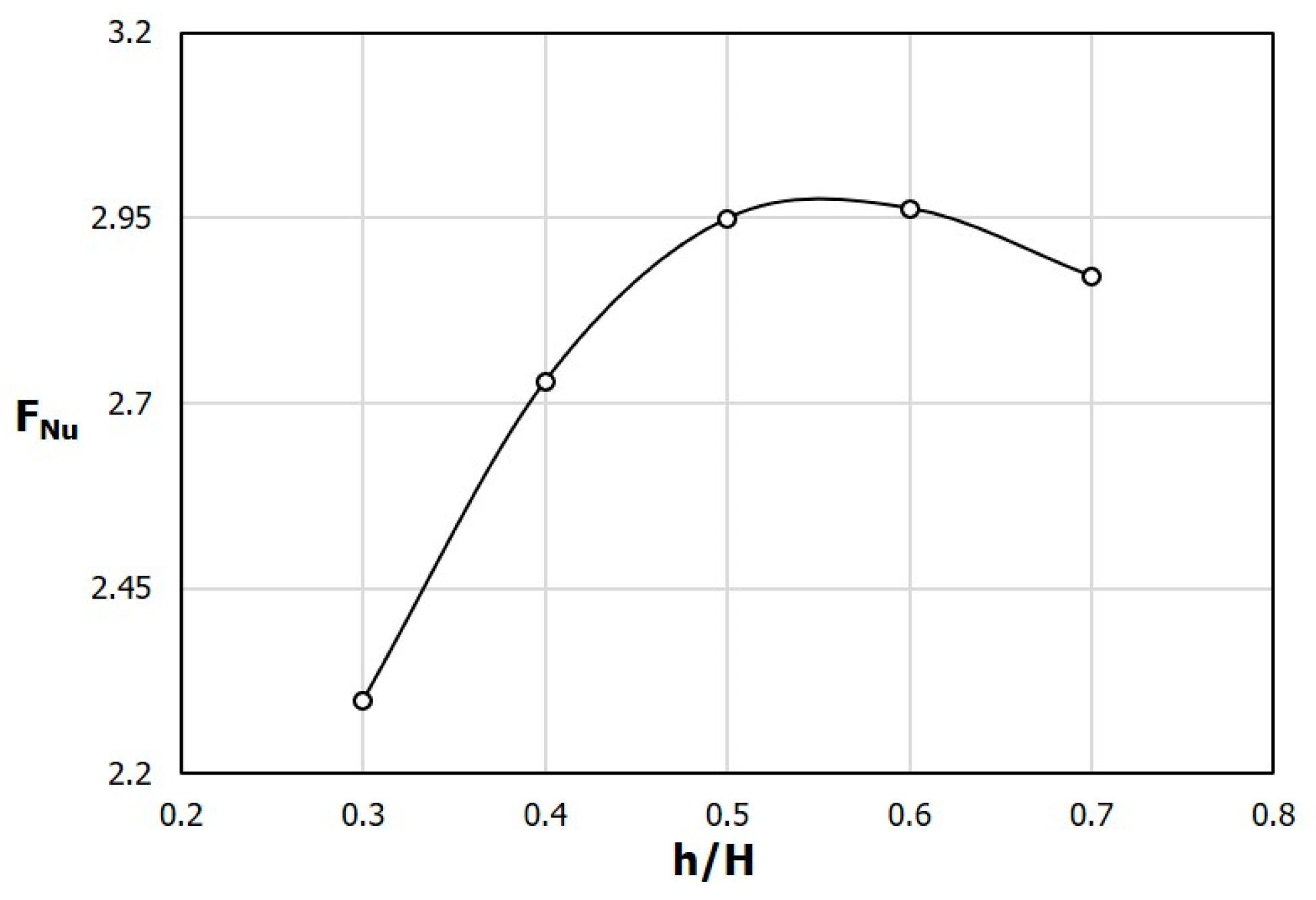Figure 6. Variation of the performance parameter related to the pressure drop (Ff) with h/H (e/W = 0.1).
Figure 6. Variation of the performance parameter related to the pressure drop (Ff) with h/H (e/W = 0.1).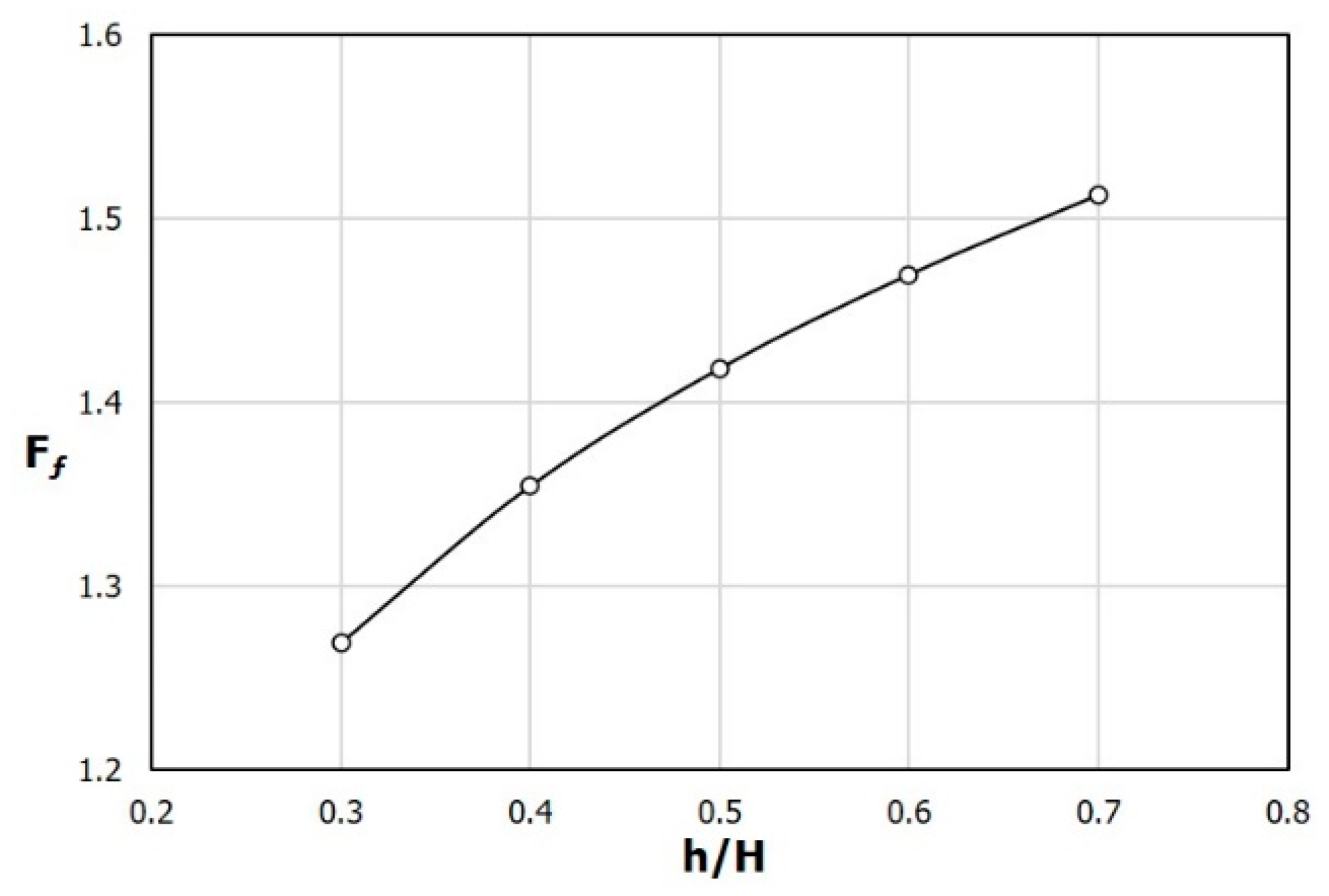Figure 7. Variation of performance factor (PF) with h/H (e/W = 0.1).
Figure 7. Variation of performance factor (PF) with h/H (e/W = 0.1).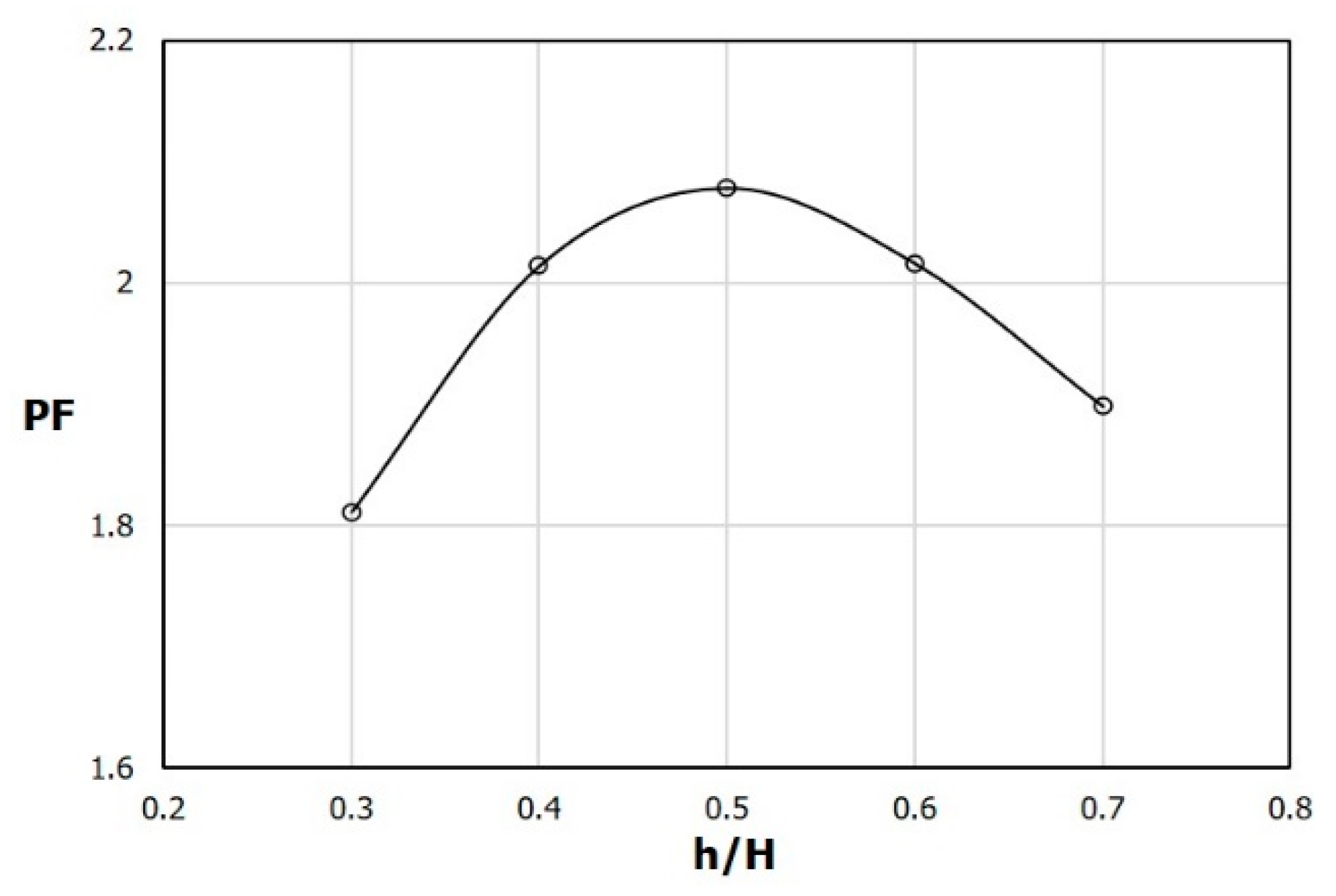Figure 8. Streamlines on x-y plane (z = 0) between fifth and seventh ribs (dark region in Figure 1b) for different h/H. (a) h/H = 0.3, (b) h/H = 0.5, (c) h/H = 0.7.
Figure 8. Streamlines on x-y plane (z = 0) between fifth and seventh ribs (dark region in Figure 1b) for different h/H. (a) h/H = 0.3, (b) h/H = 0.5, (c) h/H = 0.7.Figure 9. Nusselt number distributions on the heated surface for different h/H. (a) h/H = 0.3, (b) h/H = 0.5.
Figure 9. Nusselt number distributions on the heated surface for different h/H. (a) h/H = 0.3, (b) h/H = 0.5.Figure 10. Variation of FNu with e/W (h/H = 0.5).
Figure 10. Variation of FNu with e/W (h/H = 0.5).Figure 11. Variation of Ff with e/W (h/H = 0.5).
Figure 11. Variation of Ff with e/W (h/H = 0.5).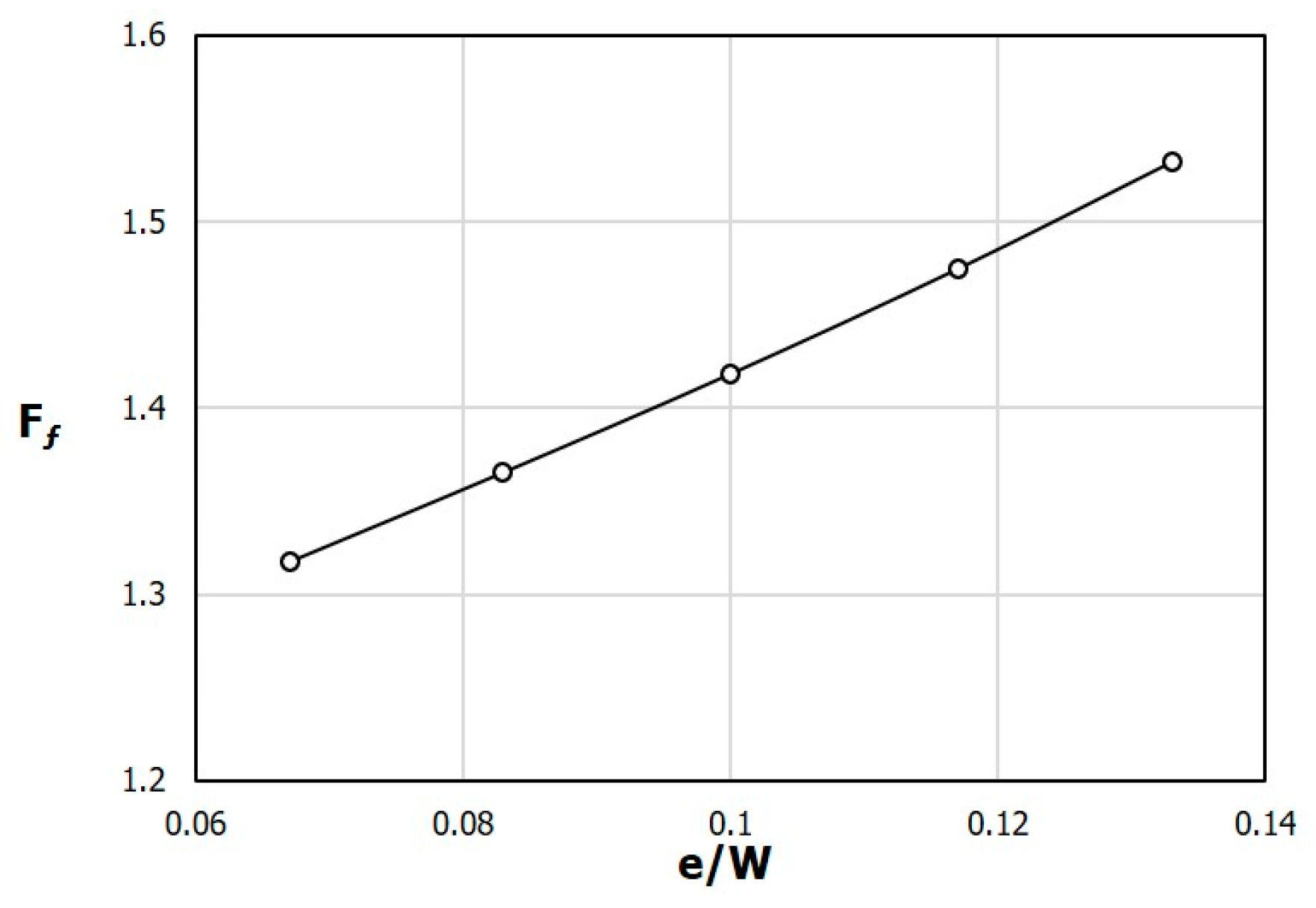Figure 12. Variation of PF with e/W (h/H = 0.5).
Figure 12. Variation of PF with e/W (h/H = 0.5).Figure 13. Streamlines on x-y plane (z = 0) between fifth and seventh ribs for different e/W. (a) e/W = 0.067, (b) e/W = 0.10, (c) e/W = 0.133.
Figure 13. Streamlines on x-y plane (z = 0) between fifth and seventh ribs for different e/W. (a) e/W = 0.067, (b) e/W = 0.10, (c) e/W = 0.133.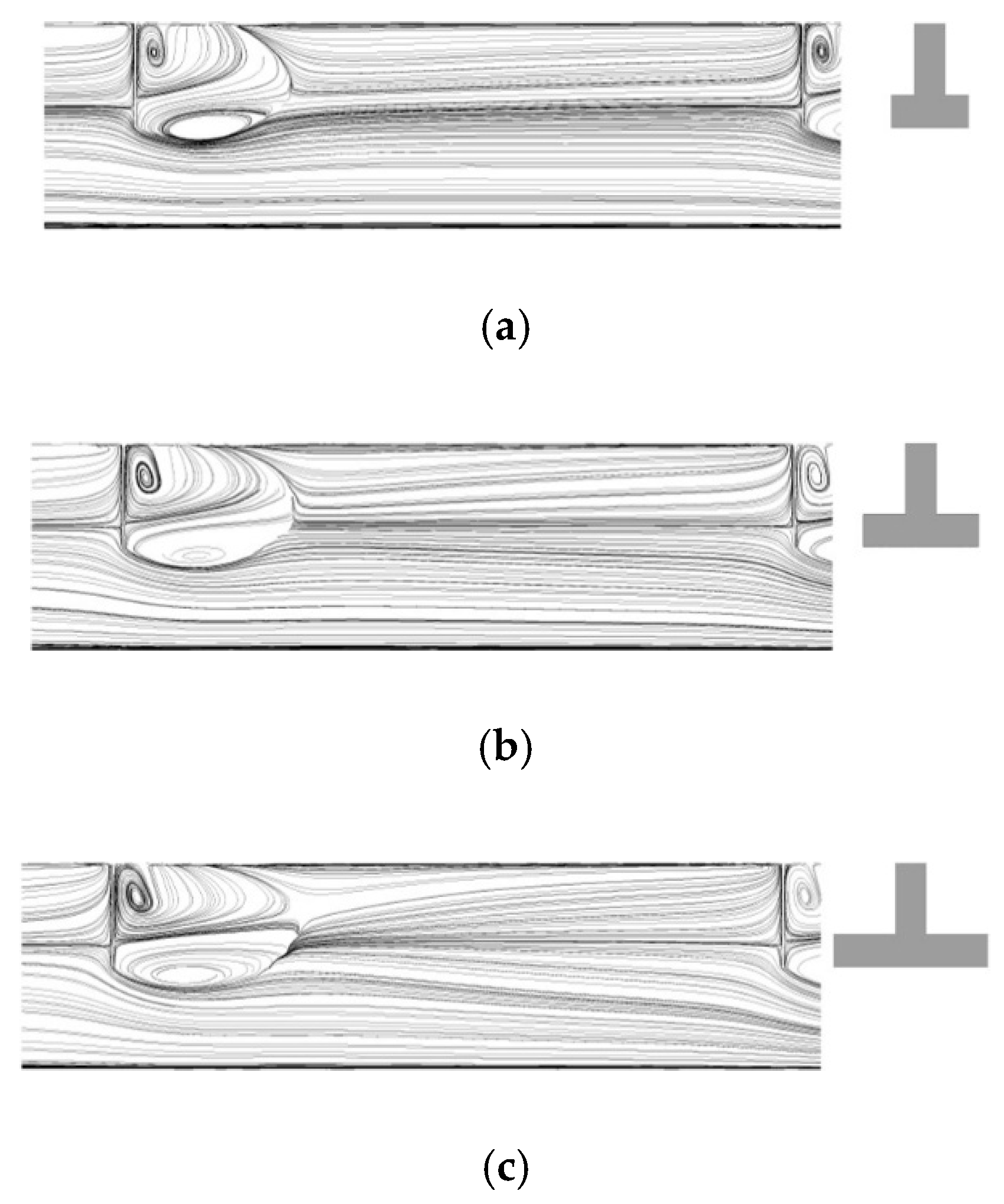Figure 14. Nusselt distributions on the heated surface between fifth and seventh ribs for different e/W. (a) e/W = 0.067; (b) e/W = 0.10; (c) e/W = 0.133.
Figure 14. Nusselt distributions on the heated surface between fifth and seventh ribs for different e/W. (a) e/W = 0.067; (b) e/W = 0.10; (c) e/W = 0.133.Figure 15. Variation of FNu with the ratio of the rib height to the rib width (h/e) (rib area = 375 mm2).
Figure 15. Variation of FNu with the ratio of the rib height to the rib width (h/e) (rib area = 375 mm2).Figure 16. Variation of Ff with h/e (rib area = 375 mm2).
Figure 16. Variation of Ff with h/e (rib area = 375 mm2).Figure 17. Variation of PF with h/e (rib area = 375 mm2).
Figure 17. Variation of PF with h/e (rib area = 375 mm2).Figure 18. Nusselt number distributions on the heated surface between fifth and seventh ribs for different h/e. (a) h/e = 0.38; (b) h/e = 0.83; (c) h/e = 1.75.
Figure 18. Nusselt number distributions on the heated surface between fifth and seventh ribs for different h/e. (a) h/e = 0.38; (b) h/e = 0.83; (c) h/e = 1.75.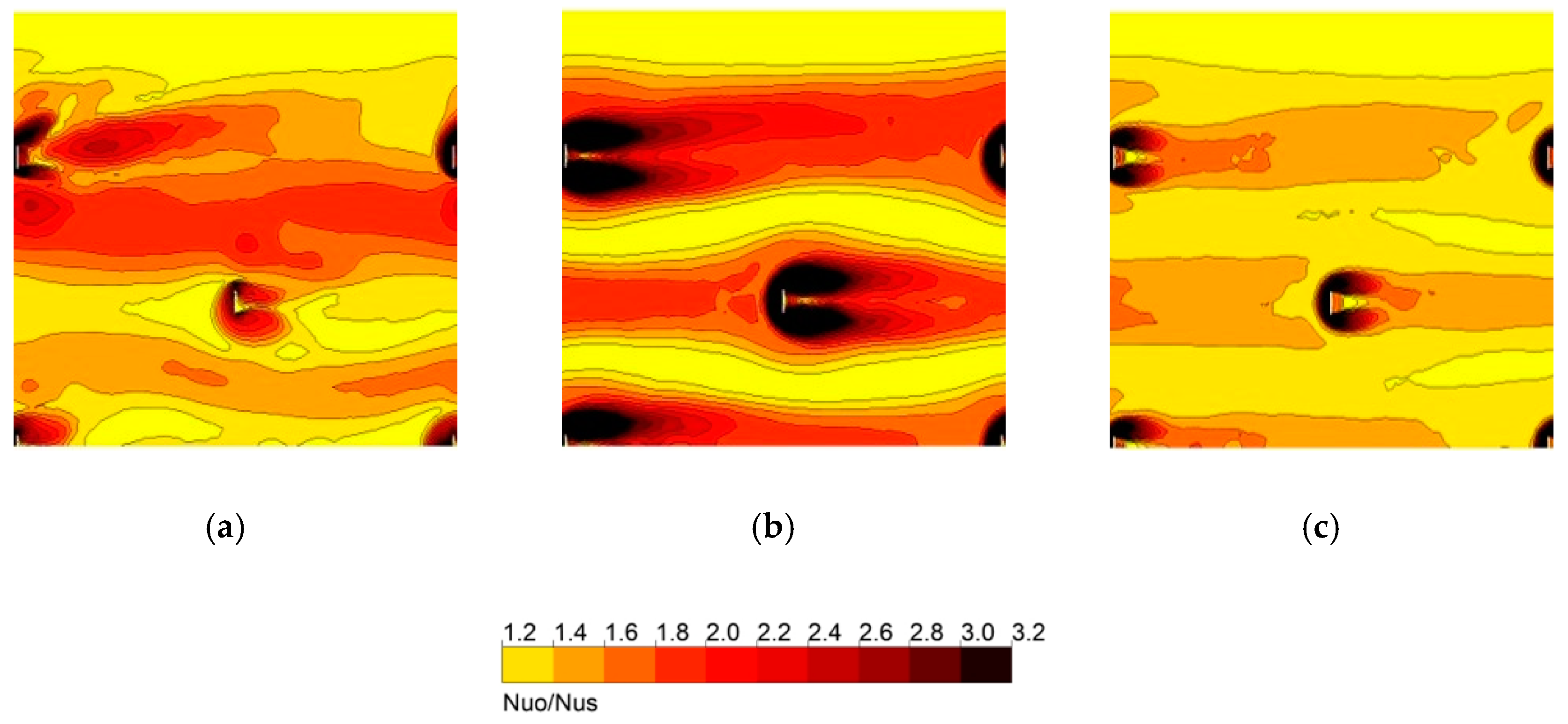Figure 19. Comparison of performance parameters between T-shaped and triangular ribs.
Figure 19. Comparison of performance parameters between T-shaped and triangular ribs.Figure 20. Comparison of PF between T-shaped and triangular ribs.
Figure 20. Comparison of PF between T-shaped and triangular ribs.Table 1. Results of grid convergence index (GCI) analysis using Richardson extrapolation.
Table 1. Results of grid convergence index (GCI) analysis using Richardson extrapolation.
Parameter Value
Number of computational cellsN1/N2/N38,071,835/3,516,156/2,336,956
Grid refinement factorr1.3
Computed Nusselt numbers
corresponding to N1, N2, N3
$ϕ$1178.137
$ϕ$2178.356
$ϕ$3180.072
Apparent orderp5.306
Extrapolated value$ϕ e x t 21$178.134
Approximate relative error$e a 21$0.123%
Extrapolated relative error$e e x t 21$0.0015%
Grid convergence index$G C I f i n e 21$0.0019%
Table 2. Ranges and reference values of geometric parameters.
Table 2. Ranges and reference values of geometric parameters.
ParameterLower LimitUpper LimitReference
h/H0.30.70.50
e/W0.0670.1330.10
h/e0.381.750.83
Table 3. Comparison of performance factor range obtained in the present study with those obtained in the previous experimental or numerical studies on obstacle shape.
Table 3. Comparison of performance factor range obtained in the present study with those obtained in the previous experimental or numerical studies on obstacle shape.
InvestigatorGeometrical Shape of ObstaclesParametersPF Range
Bekele et al. Delta-shaped ribsRe = 3400–28000
Pl/e = 1.5–5.5
e/H = 0.5–0.75
θ = 90 °
1.10–2.14
Yadav et al. Equilateral triangular sectioned ribsRe = 3800-18000
Pl/e = 7.14–35.71
e/D = 0.021–0.042
1.36–2.11
Deo et al. Multi-gap V-down ribs combined with staggered ribsRe = 4000–12000
P/e = 4–14
e/D =0.026–0.057
θ = 40°– 80 °
2.09–2.45
Gawande et al. L-shaped ribsRe = 3800–18000
P/e = 7.14–17.86
e/D = 0.042
1.62–1.90
Sawhney et al. Wavy delta wingletsRe = 4000–17300
P/H = 3–6
ϕ = 3–7
θ = 60 °
1.46–2.09
Present studyT-shaped ribsRe = 4510–22600
h/H = 0.3–0.7
e/W = 0.067–0.133
h/e =0.38–1.75
2.07–2.63
 Publisher’s Note: MDPI stays neutral with regard to jurisdictional claims in published maps and institutional affiliations.